# C program to implement MERGE sort Algorithm

In this tutorial you will learn about the C program to implement MERGE sort Algorithm and its application with practical example.

## C Program to Merge sort Algorithm

In this tutorial, we will learn to create a C program that will do Merge sort using C programming.

## Prerequisites

Before starting with this tutorial we assume that you are best aware of the following C programming topics:

• Operators in C Programming.
• Basic Input and Output function in C Programming.
• Basic C programming.
• While loop in C programming.
• For loop in C Programming.
• Creating and Using the user-defined function in C programming.

## What is Merge Sort?

In every programming language, the sorting of data is a very important factor.  In C Language. Many different techniques for sorting are available to work with but in this tutorial, we will focus on the Merge Sort Technique.

In this technique, we will divide the array into smaller chunks until there is only one element left. Then we will merge the chunks and gets a sorted array.

## Output:-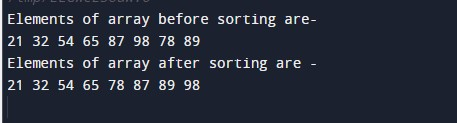In the above program, we have first declared and initialized a set variables required in the program.

• no = it will hold the size of array.
• x[]= it will hold the elements in a array.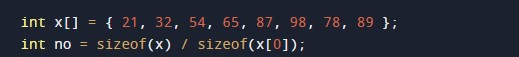Subarray sorting merging Function Body:-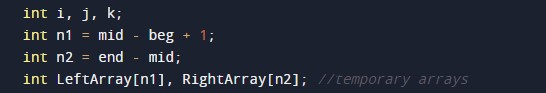• i = it will hold the integer value to control the array.
• j = it will hold the integer value to control the array.
• k= it will hold the integer value to control the array.
• LeftArray = it will hold the left array values.
• RightArray = it will hold the left array values.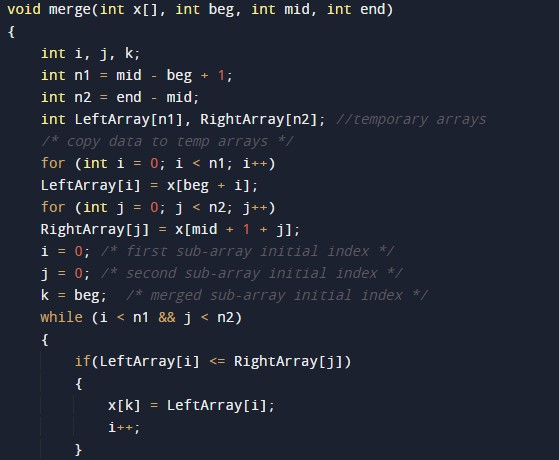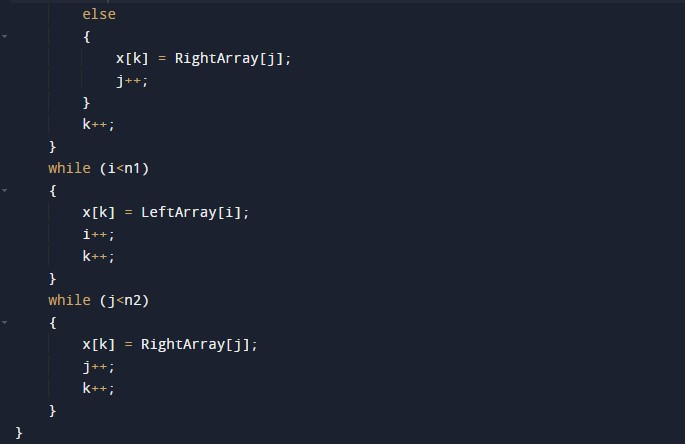Merge Sorting Function Body:-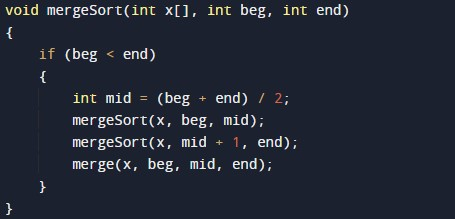Print Array Function body:-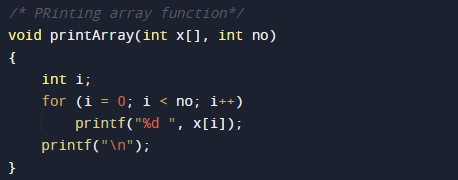The main function to perform and navigate the program cleanly.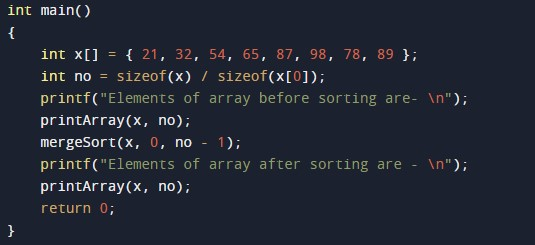In this tutorial we have learn about the C program to implement MERGE sort Algorithm and its application with practical example. I hope you will like this tutorial.